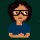Coffee Room
Discuss anything here - everything that you wish to discuss with fellow engineers.
12914 Members
Join this group to post and comment.electron1212 • Oct 15, 2008

# How Much Alcohol :P

A 20 litre vessel is filled with alcohol. Some of the alcohol is poured out into another vessel of an equal capacity, which is then completely filled by adding water. The mixture thus obtained is then poured into the first vessel to capacity. Then (20/3) litres is poured from the first vessel into the second. Both vessels now contain an equal amount of alcohol. How much alcohol was originally poured from the first vessel into the second ?

Crack this and also mention the steps you have taken.durga ch • Oct 15, 2008
initial volumn 1st vesel=20lts-filled
initial volumn 2nd vessel=20lts-empty

volumn transferred=xlts

volumn in first vessel=20-xlts
and volumn in seoncd vessel=xlts

amount of volumn of water added =20-x in second vessel

now what ever amount of seoncd vessels liquid is transferred, the volumn solution in first vessel will be = 20

but since content to be transfered is x... now the total volumn in seocnd vessel is 20-x where as volumn in vessel 1 is 20

transfering 20/3 lts to second vessel makes the volumnequal.

20-20/3=20-x

x=20/3lts

I have not considered the concentrations thoughelectron1212 • Oct 15, 2008
sorry thats not the correct answer.durga ch • Oct 15, 2008
oh okie!!😔
will give it a second go!!electron1212 • Oct 15, 2008
44+ views but still no correct answer/explanation and i thought this one was easy.:sshhh:mech_guy • Jul 8, 2009
Lets say, Alcohol transferred from first vessel to second is "V"

So, alcohol in first vessel = 20 - V
alcohol in 2nd vessel = V

Now, we add (20-V) lt of water in second vessel and mix it well.
To completely fill first vessel V lt. solution should be taken from vessel two.
Alcohol in this V lt of solution will be = V/(20/V) = V*V/20

Amount of alcohol in first vessel now = (20 - V + V*V/20) = (20 - X) in 20 lt solution
Amount of alcohol in second vessel now = (V - V*V/20) = X

where, V - (V*V/20) = X

Now (20/3) lt of solution is taken from first vessel.
Alcohol in this (20/3) lt of solution will be = (20 - X)/3

So, now alcohol left in first vessel = (20 - x) - [(20 - x)/3} = 2*(20 - X)/3
and alcohol in second vessel = X + (20 - X)/3 = (20 + 2*x)/3

equating both will give X = 5
i.e. V - (V*V/20) = 5
which gives V = 10 lts

So originally 10 lts of alcohol was transferred from first vessel to second.

RegardsSolution looks good to me, good work mech_guy.mech_guy
Lets say, Alcohol transferred from first vessel to second is "V"

So, alcohol in first vessel = 20 - V
alcohol in 2nd vessel = V

Now, we add (20-V) lt of water in second vessel and mix it well.
To completely fill first vessel V lt. solution should be taken from vessel two.
Alcohol in this V lt of solution will be = V/(20/V) = V*V/20

Amount of alcohol in first vessel now = (20 - V + V*V/20) = (20 - X) in 20 lt solution
Amount of alcohol in second vessel now = (V - V*V/20) = X

where, V - (V*V/20) = X

Now (20/3) lt of solution is taken from first vessel.
Alcohol in this (20/3) lt of solution will be = (20 - X)/3

So, now alcohol left in first vessel = (20 - x) - [(20 - x)/3} = 2*(20 - X)/3
and alcohol in second vessel = X + (20 - X)/3 = (20 + 2*x)/3

equating both will give X = 5
i.e. V - (V*V/20) = 5
which gives V = 10 lts

So originally 10 lts of alcohol was transferred from first vessel to second.

Regards
Looks fine..

-CBjhbalaji • Jul 26, 2009
need clue mate...